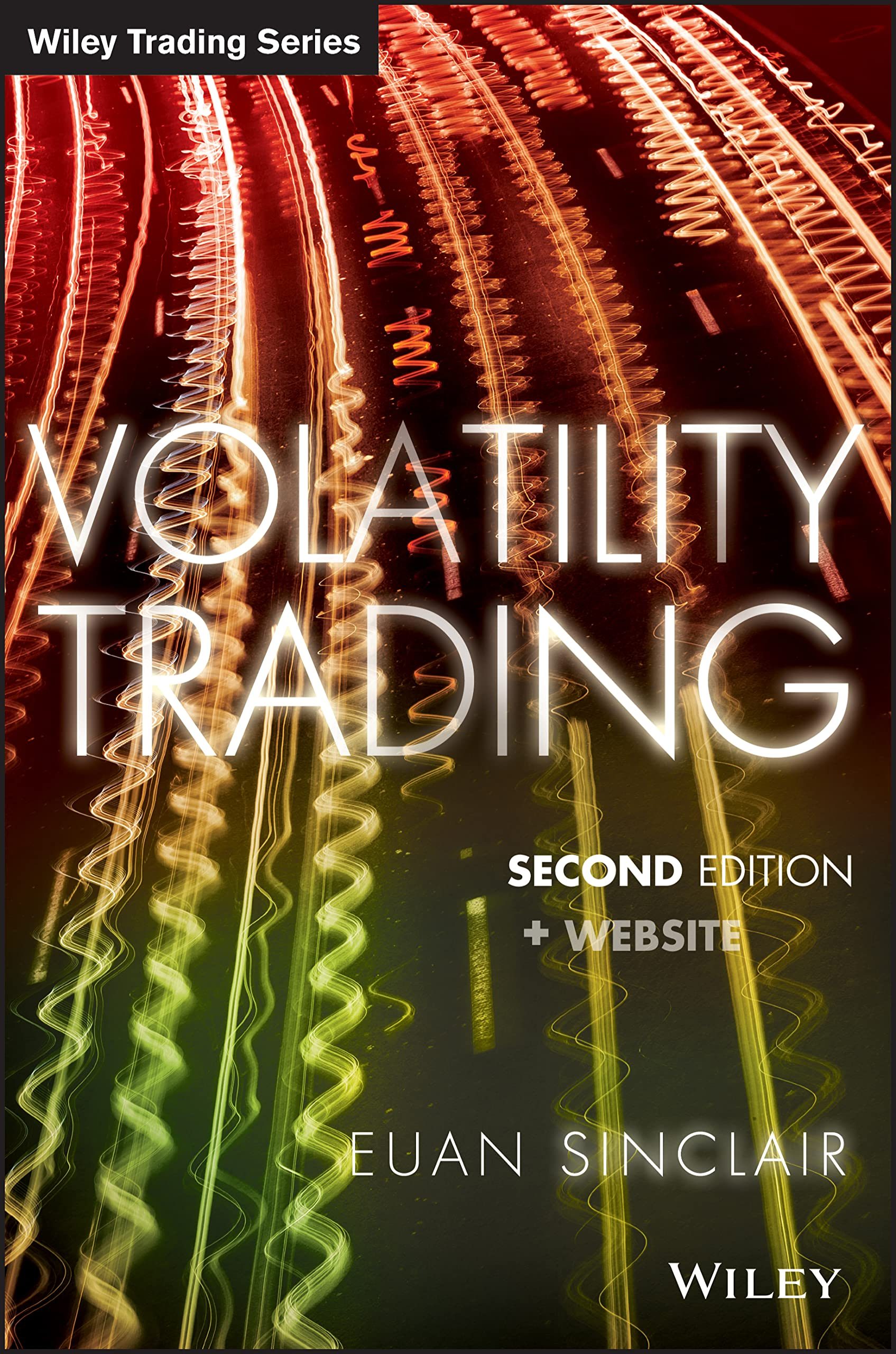A good book for an introduction to volatility from a trading perspective.

Some excerpts from Volatility Trading by Sinclair:

I am a trader. I am not a mathematician, financial engineer, or philosopher. My success is measured in profits. The tools I use and develop need only be useful. They need not be consistent, provable, profound, or even true. My approach to trading is mathematical, but I am no more interested in mathematics than a mechanic is interested in his tools. However, a certain level of knowledge, familiarity, and even respect is needed to get the most out of these tools.

This form of P/L equation is the more useful to traders, who are generally more interested in total profit than instantaneous profit. It is also easier to think about as it is linear in volatility. If we have to hold the option to expiration and realized volatility averages $\sigma$, we will also make this amount, but only on average. The “vega profit” is realized as the sum of the hedges as we rebalance our delta. The problem this presents is that the gamma is highly dependent on the moneyness of the option, which obviously changes as the underlying moves around. So the profit is highly volatile and path-dependent.

Several estimators for volatility measurement: close/close volatility, Parkinson volatility, Rogers/Satchell volatility, Garman/Klass volatility, and Yang/Zhang volatility.

Properties of historical volatility: mean reversion, volatility clustering, and seasonality effects.

List of Stylized Facts:

• Volatility is not constant. It mean-reverts, clusters, and possesses long memory.
• Large returns occur relatively frequently. These large moves have subsequent aftershocks.
• In most markets, volatility and returns have a negative correlation. This effect is asymmetric: negative returns cause volatility to rise sharply while positive returns lead to a smaller drop in volatility. This effect occurs most prominently in equity markets.
• Volatility and volume have a strong positive relationship.
• The distribution of volatility is close to log-normal.

Volatility forecasting using fundamental information:

Sridharan (2012) shows that the market underestimates volatility for firms with:

• High research and development costs.
• High cash flow volatility.
• Earnings management.

The market overestimates volatility for firms with:

• Large size.
• High return on assets.
• High leverage.

Given that there is a relationship between leverage and default, it seems that naively, we would want to be long volatility on highly levered firms. In fact though, for a firm to become highly leveraged it needs good access to the debt markets, and the bond market has a better understanding of this risk than the volatility market does.

At-the-money volatility movement dominates the movement of the implied volatility surface.

This pattern of implied volatility rising before the announcement, then dropping once the news is released, is ubiquitous.

There are a couple of interesting trade ideas mentioned here (pages 74-79).

Page 79 describes how to derive the expected absolute return (the jump) from the ATM implied volatilities of the front-month and second-month options.

The volatility attributed to the event is the difference of the front-month volatility and the forward volatility.

$jump = \sqrt{\frac{2}{\pi}} \sigma_{event}$

P/L = $vega(\sigma_{implied} - \sigma_{realized})$ is only true on average. Real P/L is strongly path dependent, and depends on the discrete hedging scheme. As a consequence, correctly predicting realized volatility is no guarantee of being profitable. Some variance in P/L can be eliminated by hedging more frequently (at a higher cost).

There are several well-known premia: